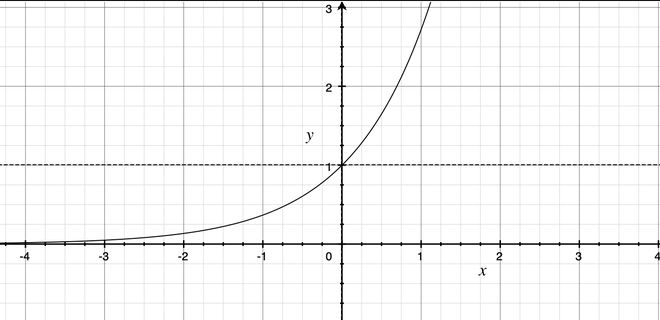# Calculate exp(x) – 1 for all elements in a given NumPy array

• Difficulty Level : Medium
• Last Updated : 27 Aug, 2021

Exponential Function (e^x) is a mathematical function that calculates e raised to the power x where e is an irrational number, approximately 2.71828183.Attention geek! Strengthen your foundations with the Python Programming Foundation Course and learn the basics.

To begin with, your interview preparations Enhance your Data Structures concepts with the Python DS Course. And to begin with your Machine Learning Journey, join the Machine Learning - Basic Level Course

It can be calculated using the numpy.exp() method. This mathematical function helps user to calculate exponential of all the elements in the input array.

Syntax: numpy.exp(arr, out, where)

Parameters:
arr : Input
out : A location into which the result is stored. If provided, it must have a shape that the
inputs broadcast to. If not provided or None, a freshly-allocated array is returned.
shape must be same as input array.
where : Boolean Value.True value means to calculate the universal functions(ufunc) at that position, False value means to leave the value in the output alone.

If a scalar is provided to the function as input then the function is applied on the scalar and another scalar is returned.

Example 1: If 3 was given as input then e^3 will returned as output.

## Python

 `import` `numpy` `n ``=` `4``print``(numpy.exp(n))` `n ``=` `5``print``(numpy.exp(n))`

Output :

```54.598150033144236
148.4131591025766```

If input is an array then function is applied element-wise. ex- np.exp([1,2,3]) is equivalent to [np.exp(1),np.exp(2),np.exp(3)]

Method 1: Iterating over array

## Python

 `# importing numpy``import` `numpy`  `arr ``=` `[``1``, ``2``, ``3``, ``4``]` `print``(``"Input : "``, arr)``for` `i ``in` `range``(``len``(arr)):``    ``arr[i] ``=` `numpy.exp(arr[i])``-``1` `print``(``"Output : "``, arr)` `arr ``=` `[``3``, ``0.3``, ``3.1``, ``2.2``]` `print``(``"Input : "``, arr)``for` `i ``in` `range``(``len``(arr)):``    ``arr[i] ``=` `numpy.exp(arr[i])``-``1` `print``(``"Output : "``, arr)`

Output:

Input :  [1, 2, 3, 4]
Output :  [1.718281828459045, 6.38905609893065, 19.085536923187668, 53.598150033144236]
Input :  [3, 0.3, 3.1, 2.2]
Output :  [19.085536923187668, 0.3498588075760032, 21.197951281441636, 8.025013499434122]

Method 2: Providing array as input to numpy.exp() function

## Python

 `# importing numpy``import` `numpy` `arr ``=` `[``1``, ``2``, ``3``, ``4``]` `print``(``"Input : "``, arr)` `arr ``=` `numpy.exp(arr)``-``1``print``(``"Output : "``, arr)`  `arr ``=` `[``3``, ``0.3``, ``3.1``, ``2.2``]` `print``(``"Input : "``, arr)` `arr ``=` `numpy.exp(arr)``-``1``print``(``"Output : "``, arr)`

Output:

Input :  [1, 2, 3, 4]
Output :  [ 1.71828183  6.3890561  19.08553692 53.59815003]
Input :  [3, 0.3, 3.1, 2.2]
Output :  [19.08553692  0.34985881 21.19795128  8.0250135 ]

My Personal Notes arrow_drop_up